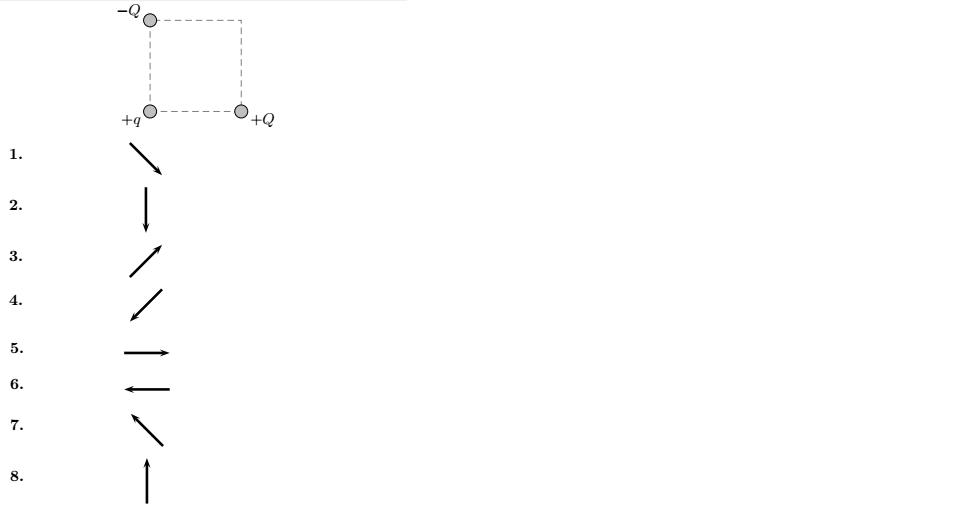🤓 Based on our data, we think this question is relevant for Professor Lai's class at TEXAS.

# Solution: Two charged particles of equal magnitude (−Q and +Q) are fixed at opposite corners of a square that lies in a plane (see figure below). A test charge +q is placed at a third corner. What is the direction of the force on the test charge due to the two other charges?

###### Problem

Two charged particles of equal magnitude (−Q and +Q) are fixed at opposite corners of a square that lies in a plane (see figure below). A test charge +q is placed at a third corner. What is the direction of the force on the test charge due to the two other charges?